# Using the Partial Products Method for Multi-Digit Multiplication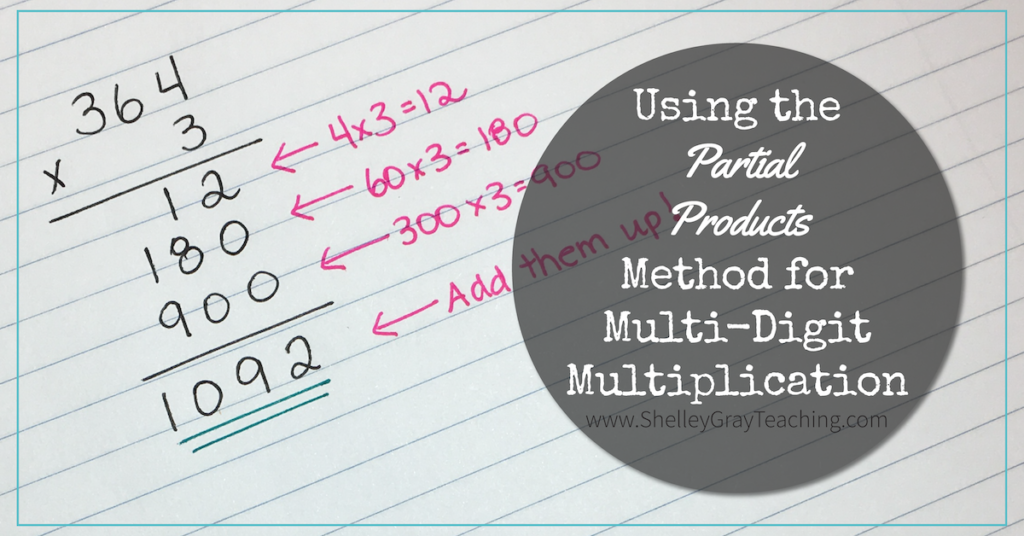Partial products is a fantastic strategy to teach as students are learning to do multi-digit multiplication. What makes it so fantastic? Well this strategy is rooted in number sense understanding. Students use place value concepts to multiply the numbers, making it a strategy that actually enhances number sense, rather than relying on a series of memorized steps.

I like this strategy as a follow up to the area model (or box/window method), which you can read more about HERE.

When we use partial products to solve a multiplication equation, we can set it up like a traditional long multiplication equation, as shown below.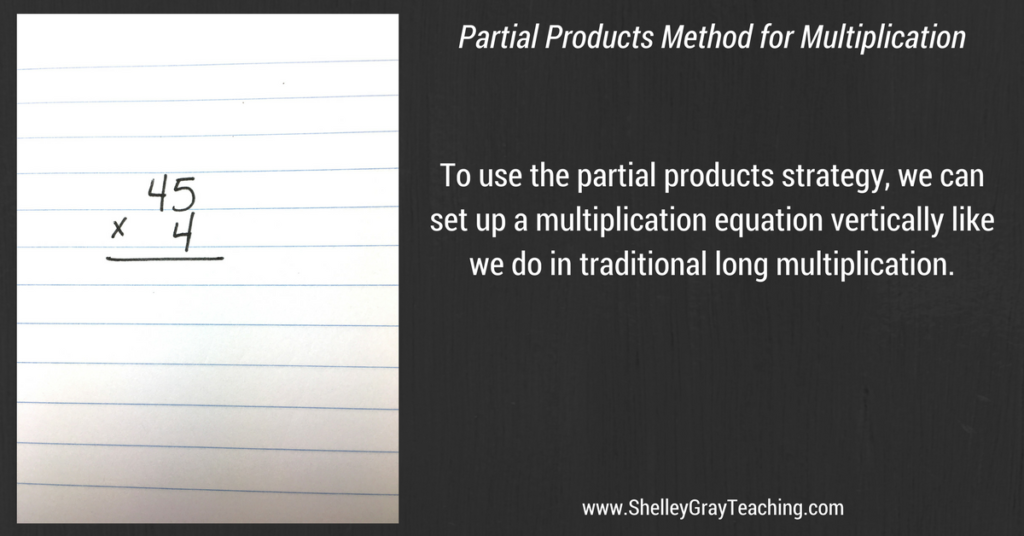Just like traditional long multiplication, we multiply the ones digit of the second factor first. In this case there is only one digit in the second factor. So first we multiply 4×5 to make 20.

Here’s where this strategy differs from traditional long multiplication. We write the entire 20 rather than using carrying as in traditional long multiplication.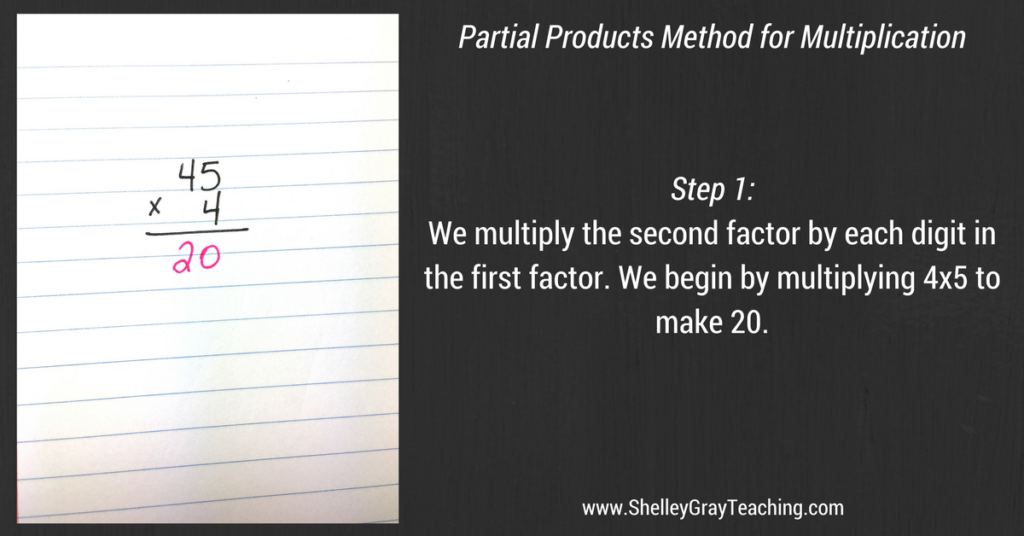Next, we multiply the 4 by the tens digit of the first factor. However in partial products we use the value of this digit. In this example, the value of the 4 in 45 is 40. So we multiply 4×40 to make 160.

We write the entire 160 beneath the 20.Now that we’ve taken care of the multiplication, we just need to add our products up, in order to make the final product. In this example, 20+160=180, so we know that 180 is the product of 45 and 4.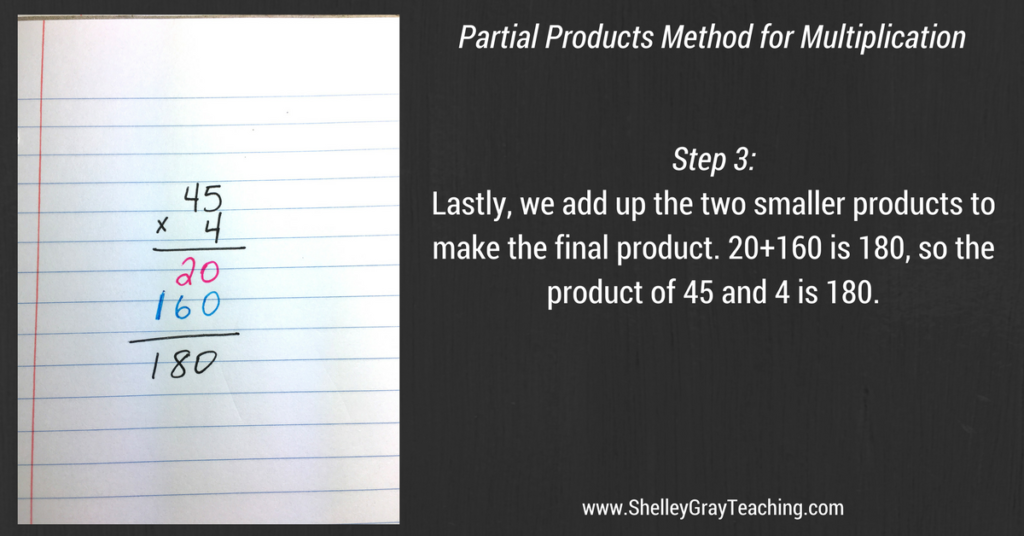When we work with more digits, we just multiply the ones first, and then the tens. The example below shows how we would solve a 2-digit by 2-digit equation. Remember that we always use the VALUE of the digits, not just the digits themselves.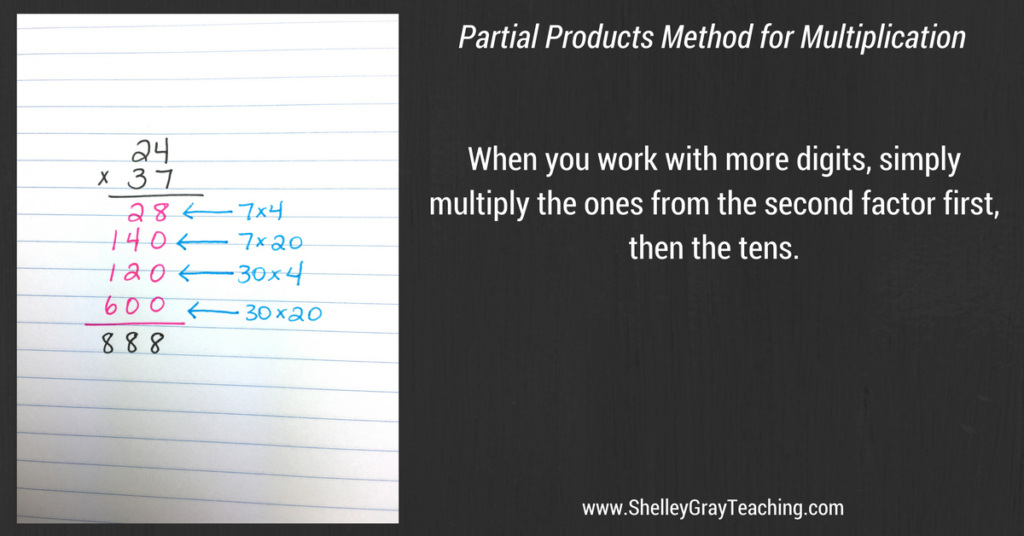NEXT STEPS